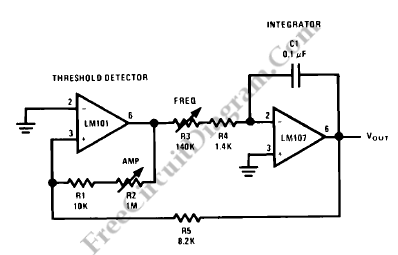# Triangular-Wave GeneratorThe circuit diagram below shows a constant amplitude triangular-wave generator. A variable frequency triangular wave whose amplitude is independent of frequency is provided by this circuit.As a reset circuit, the generator embodies an integrator as a ramp generator and a threshold detector with hysteresis. There is no further explanation is needed because the integrator has been described in a previous section. It’s a latch circuit with a large dead zone, on that case, the threshold amplifier detector is similar to a Schmidt Trigger. By using positive feedback around an operational amplifier, we can implement this function. The positive feedback network provides a voltage at the non-inverting input which is determined by the attenuation of the feedback loop and the saturation voltage amplifier when the amplifier output is in either the positive or negative saturated state. The voltage at the input of the amplifier must be caused to change polarity by an amount in excess of the amplifier input offset voltage to cause the amplifier to change states. When this is done, until the voltage at its input again reverse, the amplifier saturates in the opposite direction and remains in that state. By examining the operation with the output of the threshold detector in the positive state, the complete circuit operation may be understood. To cause a current I+ to flow, we have to apply the detector positive saturation voltage to the integrator summing junction through the combination R3 and R4.

Then, a negative-going ramp with a rate of I+/C1 volts per second until its output equals the negative trip point of the threshold detector is generated by the integrator. After that, at the integrator summing point , the threshold detector changes to the negative output state and supplies a negative current, I-. A positive-going ramp with a rate of I-/C1 volts per second is generated by the integrator until its output equals the positive trip point of the threshold detector where the detector again changes output state and the cycle repeats.

R3, R4, C1, and the positive and negative saturation voltages of the amplifier A1 determine the triangular-wave frequency. Amplitude is determined by the threshold detector saturation voltages and the ratio of R5 to the combination of R1 and R2. If the detector has equal positive and negative saturation voltages, positive and negative ramp rates are equal and positive and negative peaks are equal. If the inverting input of the threshold detector, A1, is offset with respect to ground, the output waveform may be offset with respect to ground.

If the detector is clamped with matched Zener diodes as shown in following figure, the generator may be made independent of temperature and supply voltage. If power supply impedance causes oscillation during its transition time, the detector may be compensated and the integrator should be compensated for unity-gain. For maximum symmetry, the current into the integrator should be large with respect to Ibias and offset voltage should be small with respect to Voutpeak. [Source: National Semiconductor Application Note]